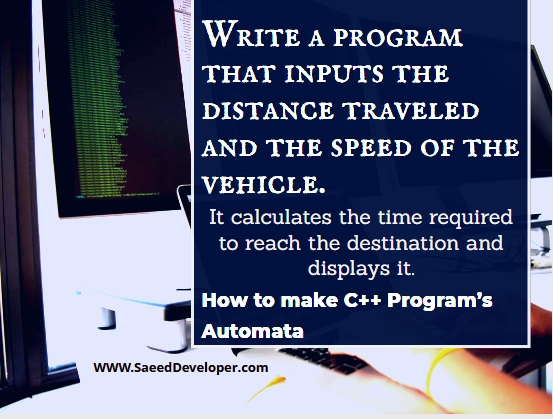# Q  Write a program that inputs the distance traveled and the speed of the vehicle.Write a program that inputs the Distance traveled and speed of the vehicle

## It calculates the time required to reach the destination and displays it.

#### It also makes the Automata of the C++ program to show the stages in the program.

In this article, I will show you how to write a program that inputs the distance traveled and speed of the vehicle and this program will also calculate the time required to reach the destination and this will also display the output of the program. In this program, I will write the whole program in the C++ programming language.
At the end of the program, I will show the whole process in the automata of the C++ program to show the stages of the program.
The best advice for you to put the whole code in your C++ compiler whichever you are using. In this way, you will get the code. After understanding all code then you should write by your self in the compiler and check the code whether its working or not. if you have any error in the code then ask me about your query.
#include<iostream.h>
#include<conio.h>
// Global variables
Int a;
Int b;
Int c;
Void take data ();
Void process data ();
Void show data ();
Void main ()
{
Take data ();
Process data ();
Show data ();
}
Void take data ()
{
Cout <<” inputs the distance traveled and speed of vehicle“;
}
Void process data ()
{
Cout <<” calculate the time required to reach the destination and displays it“;
}
Void show data ()
{

Cout << “show the result “;
}
Automata of the program

[caption id="attachment_116" align="alignnone" width="720"]write a program that inputs the distance traveled and the speed of the vehicle[/caption]
 2
a                       b                           c
 1
 3
 4

a     1’2 =   take the inputs from the user.
b     2,3 = process . calculates the time required to reach the destination
c     3’4 = show data / result
1 is the initial state
4 is the ending state

#### How was the my this solution?

Give me feedback on how you find this solution to the C++ program. I would like to see your comments on this C++ program. If you want more answers to the problem let me know I will put more. Thanks in anticipation.
See the related articles to the post write a program that inputs the distance traveled and speed of the vehicle